# 1.6 Add and subtract fractions  (Page 2/4)

 Page 2 / 4

When finding the equivalent fractions needed to create the common denominators, there is a quick way to find the number we need to multiply both the numerator and denominator. This method works if we found the LCD by factoring into primes.

Look at the factors of the LCD and then at each column above those factors. The “missing” factors of each denominator are the numbers we need.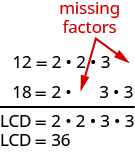In [link] , the LCD, 36, has two factors of 2 and two factors of $3.$

The numerator 12 has two factors of 2 but only one of 3—so it is “missing” one 3—we multiply the numerator and denominator by 3.

The numerator 18 is missing one factor of 2—so we multiply the numerator and denominator by 2.

We will apply this method as we subtract the fractions in [link] .

Subtract: $\frac{7}{15}-\phantom{\rule{0.2em}{0ex}}\frac{19}{24}.$

## Solution

Do the fractions have a common denominator? No, so we need to find the LCD.

 Find the LCD. $\phantom{\rule{5em}{0ex}}$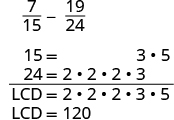Notice, 15 is “missing” three factors of 2 and 24 is “missing” the 5 from the factors of the LCD. So we multiply 8 in the first fraction and 5 in the second fraction to get the LCD. Rewrite as equivalent fractions with the LCD.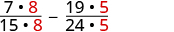Simplify.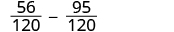Subtract. $\phantom{\rule{2em}{0ex}}-\phantom{\rule{0.2em}{0ex}}\frac{39}{120}$ Check to see if the answer can be simplified. $\phantom{\rule{2em}{0ex}}-\phantom{\rule{0.2em}{0ex}}\frac{13\cdot 3}{40\cdot 3}$ Both 39 and 120 have a factor of 3. Simplify. $\phantom{\rule{2em}{0ex}}-\phantom{\rule{0.2em}{0ex}}\frac{13}{40}$

Do not simplify the equivalent fractions! If you do, you’ll get back to the original fractions and lose the common denominator!

Subtract: $\frac{13}{24}-\phantom{\rule{0.2em}{0ex}}\frac{17}{32}.$

$\frac{1}{96}$

Subtract: $\frac{21}{32}-\phantom{\rule{0.2em}{0ex}}\frac{9}{28}.$

$\frac{75}{224}$

In the next example, one of the fractions has a variable in its numerator. Notice that we do the same steps as when both numerators are numbers.

Add: $\frac{3}{5}+\frac{x}{8}.$

## Solution

The fractions have different denominators.

 $\phantom{\rule{2em}{0ex}}$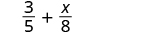Find the LCD. $\phantom{\rule{4em}{0ex}}$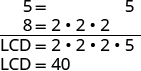Rewrite as equivalent fractions with the LCD. $\phantom{\rule{2em}{0ex}}$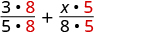Simplify. $\phantom{\rule{2em}{0ex}}$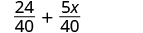Add. $\phantom{\rule{2em}{0ex}}$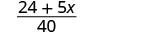Remember, we can only add like terms: 24 and 5 x are not like terms.

Add: $\frac{y}{6}+\frac{7}{9}.$

$\frac{9y+42}{54}$

Add: $\frac{x}{6}+\frac{7}{15}.$

$\frac{15x+42}{135}$

We now have all four operations for fractions. [link] summarizes fraction operations .

 Fraction Multiplication Fraction Division $\frac{a}{b}·\frac{c}{d}=\frac{ac}{bd}$ Multiply the numerators and multiply the denominators $\frac{a}{b}÷\frac{c}{d}=\frac{a}{b}·\frac{d}{c}$ Multiply the first fraction by the reciprocal of the second. Fraction Addition Fraction Subtraction $\frac{a}{c}+\frac{b}{c}=\frac{a+b}{c}$ Add the numerators and place the sum over the common denominator. $\frac{a}{c}-\phantom{\rule{0.2em}{0ex}}\frac{b}{c}=\frac{a-b}{c}$ Subtract the numerators and place the difference over the common denominator. To multiply or divide fractions, an LCD is NOT needed. To add or subtract fractions, an LCD is needed.

Simplify: $\frac{5x}{6}-\phantom{\rule{0.2em}{0ex}}\frac{3}{10}$ $\frac{5x}{6}·\frac{3}{10}.$

## Solution

First ask, “What is the operation?” Once we identify the operation that will determine whether we need a common denominator. Remember, we need a common denominator to add or subtract, but not to multiply or divide.

1. What is the operation? The operation is subtraction.
$\begin{array}{cccccc}\text{Do the fractions have a common denominator? No.}\hfill & & & & & \hfill \frac{5x}{6}-\phantom{\rule{0.2em}{0ex}}\frac{3}{10}\hfill \\ \\ \\ \text{Rewrite each fraction as an equivalent fraction with the LCD.}\hfill & & & & & \hfill \begin{array}{c}\frac{5x·5}{6·5}-\phantom{\rule{0.2em}{0ex}}\frac{3·3}{10·3}\hfill \\ \phantom{\rule{0.6em}{0ex}}\frac{25x}{30}-\phantom{\rule{0.2em}{0ex}}\frac{9}{30}\hfill \end{array}\hfill \\ \\ \\ \begin{array}{c}\text{Subtract the numerators and place the difference over the}\hfill \\ \text{common denominators.}\hfill \end{array}\hfill & & & & & \hfill \frac{25x-9}{30}\hfill \\ \\ \\ \begin{array}{c}\text{Simplify, if possible There are no common factors.}\hfill \\ \text{The fraction is simplified.}\hfill \end{array}\hfill & & & & & \end{array}$
2. What is the operation? Multiplication.
$\begin{array}{cccccc}& & & & & \hfill \phantom{\rule{1em}{0ex}}\frac{5x}{6}·\frac{3}{10}\hfill \\ \\ \\ \begin{array}{c}\text{To multiply fractions, multiply the numerators and multiply}\hfill \\ \text{the denominators.}\hfill \end{array}\hfill & & & & & \hfill \phantom{\rule{1em}{0ex}}\frac{5x·3}{6·10}\hfill \\ \\ \\ \begin{array}{c}\text{Rewrite, showing common factors.}\hfill \\ \text{Remove common factors.}\hfill \end{array}\hfill & & & & & \hfill \phantom{\rule{1em}{0ex}}\frac{\overline{)5}x·\overline{)3}}{2·\overline{)3}·2·\overline{)5}}\hfill \\ \\ \\ \text{Simplify.}\hfill & & & & & \hfill \phantom{\rule{1em}{0ex}}\frac{x}{4}\hfill \end{array}$

Notice we needed an LCD to add $\frac{5x}{6}-\phantom{\rule{0.2em}{0ex}}\frac{3}{10},$ but not to multiply $\frac{5x}{6}·\frac{3}{10}.$

Aziza is solving this equation-2(1+x)=4x+10
No. 3^32 -1 has exactly two divisors greater than 75 and less than 85 what is their product?
x^2+7x-19=0 has Two solutions A and B give your answer to 3 decimal places
3. When Jenna spent 10 minutes on the elliptical trainer and then did circuit training for20 minutes, her fitness app says she burned 278 calories. When she spent 20 minutes onthe elliptical trainer and 30 minutes circuit training she burned 473 calories. How manycalories does she burn for each minute on the elliptical trainer? How many calories doesshe burn for each minute of circuit training?
.473
Angelita
?
Angelita
John left his house in Irvine at 8:35 am to drive to a meeting in Los Angeles, 45 miles away. He arrived at the meeting at 9:50. At 3:30 pm, he left the meeting and drove home. He arrived home at 5:18.
p-2/3=5/6 how do I solve it with explanation pls
P=3/2
Vanarith
1/2p2-2/3p=5p/6
James
Cindy
4.5
Ruth
is y=7/5 a solution of 5y+3=10y-4
yes
James
Cindy
Lucinda has a pocketful of dimes and quarters with a value of $6.20. The number of dimes is 18 more than 3 times the number of quarters. How many dimes and how many quarters does Lucinda have? Rhonda Reply Find an equation for the line that passes through the point P ( 0 , − 4 ) and has a slope 8/9 . Gabriel Reply is that a negative 4 or positive 4? Felix y = mx + b Felix if negative -4, then -4=8/9(0) + b Felix -4=b Felix if positive 4, then 4=b Felix then plug in y=8/9x - 4 or y=8/9x+4 Felix Macario is making 12 pounds of nut mixture with macadamia nuts and almonds. macadamia nuts cost$9 per pound and almonds cost $5.25 per pound. how many pounds of macadamia nuts and how many pounds of almonds should macario use for the mixture to cost$6.50 per pound to make?
Nga and Lauren bought a chest at a flea market for $50. They re-finished it and then added a 350 % mark - up Makaila Reply$1750
Cindy
the sum of two Numbers is 19 and their difference is 15
2, 17
Jose
interesting
saw
4,2
Cindy
Felecia left her home to visit her daughter, driving 45mph. Her husband waited for the dog sitter to arrive and left home 20 minutes, or 13 hour later. He drove 55mph to catch up to Felecia. How long before he reaches her?
hola saben como aser un valor de la expresión
NAILEA
integer greater than 2 and less than 12
2 < x < 12
Felix
I'm guessing you are doing inequalities...
Felix
Actually, translating words into algebraic expressions / equations...
Felix
hi
Darianna
hello
Mister
Eric here
Eric
6
Cindy

#### Get Jobilize Job Search Mobile App in your pocket Now!By Nick SwainBy OpenStaxBy David CoreyBy OpenStaxByBy Sebastian Sieczko...By Richley CrapoBy OpenStaxBy Angelica LitoBy Marion Cabalfin